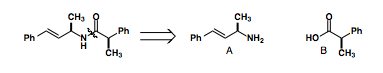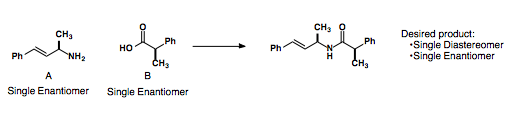# Synthetic Efficiency

$$\newcommand{\vecs}{\overset { \rightharpoonup} {\mathbf{#1}} }$$ $$\newcommand{\vecd}{\overset{-\!-\!\rightharpoonup}{\vphantom{a}\smash {#1}}}$$$$\newcommand{\id}{\mathrm{id}}$$ $$\newcommand{\Span}{\mathrm{span}}$$ $$\newcommand{\kernel}{\mathrm{null}\,}$$ $$\newcommand{\range}{\mathrm{range}\,}$$ $$\newcommand{\RealPart}{\mathrm{Re}}$$ $$\newcommand{\ImaginaryPart}{\mathrm{Im}}$$ $$\newcommand{\Argument}{\mathrm{Arg}}$$ $$\newcommand{\norm}{\| #1 \|}$$ $$\newcommand{\inner}{\langle #1, #2 \rangle}$$ $$\newcommand{\Span}{\mathrm{span}}$$ $$\newcommand{\id}{\mathrm{id}}$$ $$\newcommand{\Span}{\mathrm{span}}$$ $$\newcommand{\kernel}{\mathrm{null}\,}$$ $$\newcommand{\range}{\mathrm{range}\,}$$ $$\newcommand{\RealPart}{\mathrm{Re}}$$ $$\newcommand{\ImaginaryPart}{\mathrm{Im}}$$ $$\newcommand{\Argument}{\mathrm{Arg}}$$ $$\newcommand{\norm}{\| #1 \|}$$ $$\newcommand{\inner}{\langle #1, #2 \rangle}$$ $$\newcommand{\Span}{\mathrm{span}}$$$$\newcommand{\AA}{\unicode[.8,0]{x212B}}$$

### Linear Synthesis

We can determine the overall yield of a linear synthetic sequence by multiplying the yields of each individual step. For example...

Steps Yield/Step (%) Overall yield (%)
10 70 2.8
15 70 0.5
10 90 35
10 95 60
100 95 0.0059

As you can see, the overall yield of a linear sequence of steps quickly drops off especially when the yields of individual steps are below 90%. Even when all of the yields of a series of reactions are above 90%, the overall yield still drops, albeit at a slower rate.

### Convergent Synthesis

To increase the efficiency of a synthetic route, we can try to break target molecules into precursors of roughly the same size and complexity. In this way we can cut down the longest linear sequence and increase our overall yield of the process.In the route above, there are still 10 overall steps in the synthesis, but our longest linear sequence (LLS) is only 6 steps. We have increased our yield to 12 %, significantly higher than the 2.8 % we would have recovered from a linear synthesis of the target.

#### Stipulations to Convergent Synthesis

If we are targeting the Amide seen below, we would disconnect the amide bond to form intermediates A and B.If we combine a racemic mixture of these intermediates, we end up with a statistical distribution of the four possible stereoisomers.So while convergent routes are the most efficient, they require that each intermediate in the synthetic pathway be either achiral or a optically pure.## Green Chemistry

Synthetic Efficiency is shared under a CC BY-NC-SA 4.0 license and was authored, remixed, and/or curated by LibreTexts.